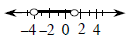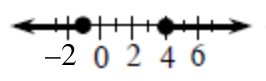### Home > CCA2 > Chapter 4 > Lesson 4.2.1 > Problem4-66

4-66.

Solve the following inequalities and draw a number line graph to represent each solution.

1. $| 2 x + 3 | < 5$

Solve the corresponding equations to determine the boundary points.

$2x+3=5$
$2x+3=−5$

Solving yields $x=−4$ or $x=1$.
Plot these points on a number line.

Test a point in each region on the number line in the original inequality. Highlight the region(s) where the test point makes the inequality true.1. $| 2 x + 3 | \geq 5$

See the steps in part (a).

The boundary points in part (b) are the same as the ones in part (a). When you test a value between the boundary points you get a false statement.
$|2(0)+3|\ge5→3\ge5$

1. $| 2 x - 3 | < 5$

See the steps in part (a).

1. $| 2 x - 3 | \geq 5$1. $| 3 - 2 x | < 5$

1. $| 3 - 2 x | \geq 5$

1. Describe any relationships you see among these six problems.

How are the graphs of the 'less than' inequalities related to the graphs of the 'greater than or equal to' inequalities?